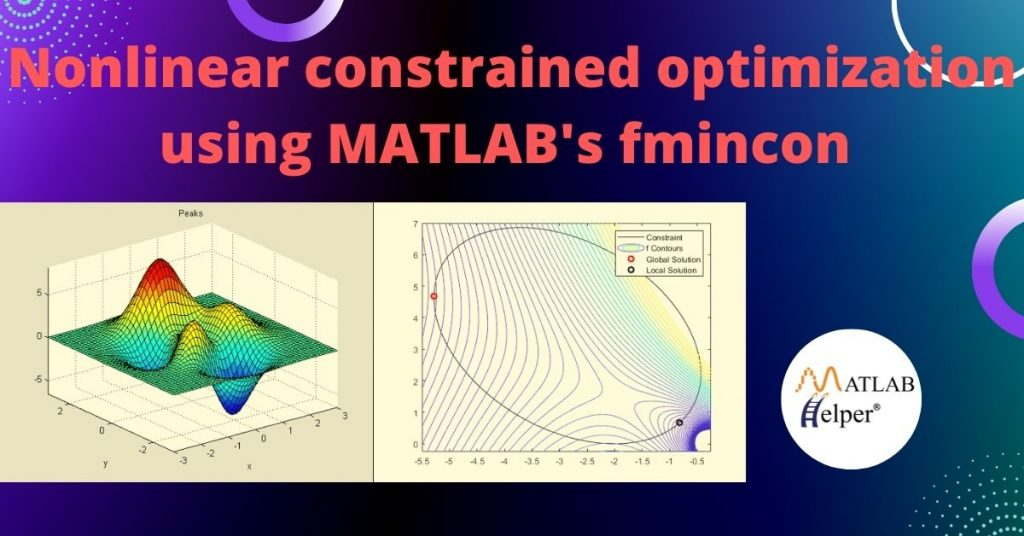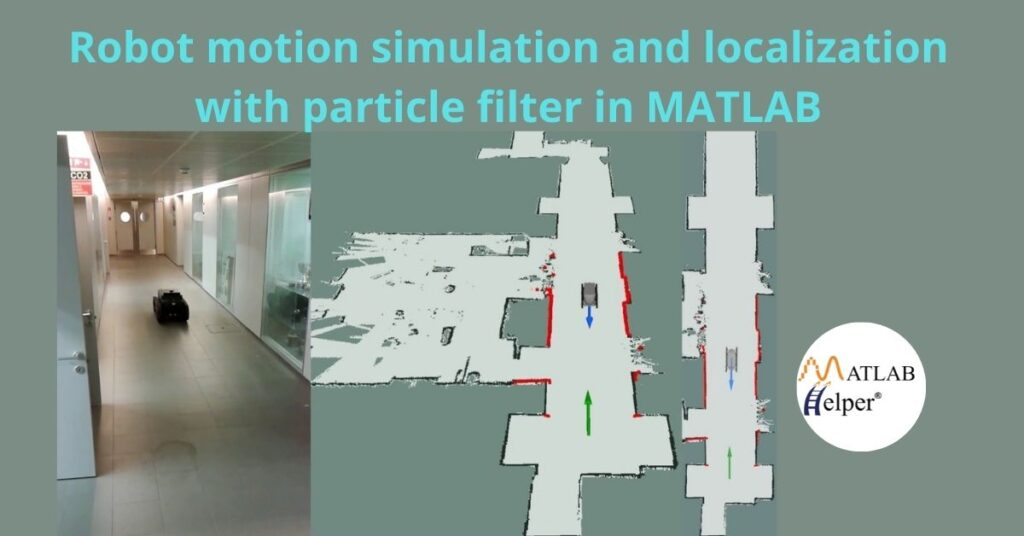## Nonlinear constrained optimization using MATLAB’s fmincon

Because of the pervasiveness of nonlinearities in modeling practical objective functions and constraints, it is essential to study the methods of solving nonlinear optimization problems with equality and/or inequality constraints. Learn how to solve them using MATLAB’s fmincon solver and graphically interpret the optimal solutions.## Robot motion simulation and localization with particle filter in MATLAB

Have you ever thought about how you could estimate the position and orientation of a mobile robot with range sensors in a known map? We can quickly and accurately achieve this using a particle filter to localize the robot. Visualize this simulation in MATLAB using GUI and get an intuitive understanding of how the particle filter work